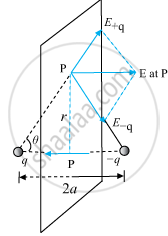# Answer the Following Question. Derive an Expression for the Electric Field at Any Point on the Equatorial Line of an Electric Dipole. - Physics

Derive an expression for the electric field at any point on the equatorial line of an electric dipole.

#### Solution

Electric field for points on the equator planeThe magnitude of the electric field due to two charges +q and –q is

E_(+q) = q/(4piε_0)1/(r^2 + a^2)

E_(-q) = q/(4piε_0)1/(r^2 + a^2)

E_(+q) = E_(-q)

The directions of E_(+q) and E_(-q), are as shown in the figure. The components normal to the dipole axis cancel away. The components along the dipole axis add up.

Total electric field, E = -(E_(+q) + E_(-q)) costheta  hatp ......(Negative sign shows that the field is opposite to hatp)

E = -(2qa)/(4piε_0(r^2 + a^2)^(3/2))hatp       ...(iii)

At large distance (r >> a), this reduces to,

E = -(2qa)/(4piε_0r^3)hatp

vecp = q xx 2vecahatp

E = -vecp/(4piε_0r^3)    ....(r >> a)

Concept: Electric Dipole
Is there an error in this question or solution?
2018-2019 (March) 55/1/3

Share